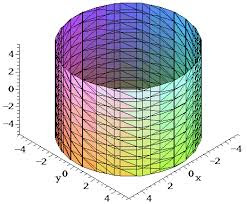## Thursday, August 17, 2017

### Unizor - Double Integrals - Circular Base

Notes to a video lecture on http://www.unizor.com

Double Integrals -
Circular Base

Previous lecture introduced a concept of a double integral as a volume of a solid with a rectangular base.
In particular, we came to a conclusion that in this case we can integrate, first, by one of the argument and then by another or, equally well, in an opposite order. Rectangular base of a solid allowed such a freedom.

In this lecture we will consider a solid with a circular base and, which is important, the technique of calculating the volume of this solid would be valid for many other types of bases, not necessarily circular. Another important point is that the integration in one order cannot be performed in another order without modifications.

Consider the following problem.
Given a smooth function of two variables f(x, y) with non-negative values (that is, f(x, y) ≥ 0), defined on an area bounded by a circle of a radius R with a center at the origin of coordinates, and represented by a surface in (X,Y,Z) coordinate space.Our task is to find the "volume of a solid" - the measure of a part of coordinate space bounded on the top by a surface representing this function, on the bottom - by X,Y-plane and sides forming a cylindrical surface based on a circle described above, rising on a height determined by the values of our function on the circle's border.

We will follow the same logic as in a case of a rectangular base.
The process of approximation starts with dividing our circular base into many small rectangles by lines x=xi (where i∈[0,M], x0=−RxM=R) and y=yj (where j∈[0,N], y0=−RyN=R) and constructing rectangular parallelepipeds on the base of these small rectangles with the height equaled to a value of function f(x, y) in the corner of each base rectangle with largest coordinates x and y ("top right corner").

If point (xi, yj) represents the coordinates of a "top right" corner of a small base rectangle and Δxi=xi−xi−1, Δyj=yj−yj−1are sides of this small rectangle, the volume of a constructed rectangular parallelepiped equals to
ΔVi,j = f(xi, yjΔxi·Δyj

The combined volume of all these parallelepipeds approximates the volume of our solid. With increasing number of small base rectangles and, correspondingly, decreasing their dimensions we assume that there is a limit of the combined volume of all these parallelepipeds.
It can be proven that under certain conditions of smoothness of our function f(x, y) the limit does exist and is unique, regardless of the way how exactly we divide the domain of our function into small rectangles, as long as their dimensions are converging to zero (or, more precisely, the dimensions of the largest among them converges to zero).

Now let's consider how we can summarize the volumes of all parallelepipeds constructed on the basis of small rectangles. We cannot summarize all xi and yj, as we did in a case of a rectangular base, because many of them will go outside of a circle where the function is undefined.
Instead, let's use the following logic. We will choose one of the arguments, let's say x, as the "primary" and will change it from x0=−R to xM=R, that is through all values of xi. For each chosen x=xi we will choose only those values of y that fall within a circle of radius R, that is from −√R²−x² to R²−x².

Therefore, while the limits of summation by "primary" argument x are all its values from x0=−R to xM=R, the limits of summation by "secondary" argument y depend on the value of "primary" x as specified above.
This dependence of the limits of summation (and, subsequently, integration) by secondary argument on the value of the primary one is the only difference of this case of circular base from the previously considered rectangular base.

Decreasing the intervals between division points by both arguments and going to a limit, we will have, firstly, an integral by a primary argument x in limits from −R to R and, for each value of x we will have to integrate by a secondary argument y in limits that depend on the value of primary argument x - from −√R²−x² to R²−x².

The final formula would look like this:
−RR [∫−√R²−x²R²−x² f(x, y) dy] dx

Let's use this formula for calculating the volume of a cylinder with f(x, y)=H - the height of a cylinder.
According to the rules of geometry, the volume of a cylinder equals to a product of an area of a base by its height, so we have to get the answer V=πR²H.
Let's check if we get it through integration.

−RR [∫−√R²−x²R²−x² dy] dx = −RR [2H√R²−x²] dx
Substitute
x=R·sin(t),
where −π/2 ≤ t ≤ π/2
Then R²−x² = R·cos(t),
dx=R·cos(t) dt,
getting
−π/2π/2[2HRcos(t)]R·cos(t)dt = 2HR²−π/2π/2cos²(t)dt

To simplify it further, use the trigonometric identity
cos(2t) = cos²(t)−sin²(t) =
= 2cos²(t) −1

from which we derive
cos²(t) = [cos(2t)+1]/2
Our integral now looks like this
2HR²−π/2π/2[cos(2t)+1]/2 dt =
HR²−π/2π/2[cos(2t)+1] dt =
HR²−π/2π/2cos(2t) dt + HR²−π/2π/2 dt
Indefinite integral (anti-derivative) of cos(2t) is (1/2)·sin(2t).
Therefore, the first integral in this sum equals to
−π/2π/2cos(2t) dt = (1/2)·[sin(2π/2)−sin(−2π/2)] = (1/2)·[sin(π)−sin(−π)] = 0

The second integral in a sum above equals to
−π/2π/2 dt = π/2 − (−π/2) = π
Therefore, the result of integration is
HR²·π = πR²H which corresponds to results from geometry.